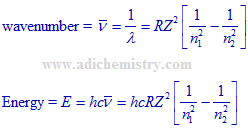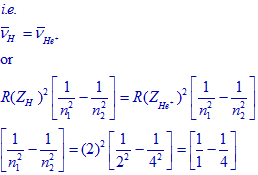# SPECTRAL LINES - HYDROGEN ATOM - ELECTRONIC TRANSITIONS IIT JEE - NEET

## The Hα spectral line in Lyman series of hydrogen atomic spectrum is formed due to an electronic transition in hydrogen atom. The energies associated with the electron in each of the orbits involved in the transition (in kCal mol-1) are:

#### (Eamcet - 2008-E)

a) -313.6, –34.84

b) -313.6, -78.4

c) -78.4, -34.84

d) -78.4, -19.6

Logic:

Energy of a quantum level or orbit in hydrogen atom in kCal mol-1 = -313.6/n2

Hα line in Lyman series of hydrogen atomic spectrum corresponds to n1= 1 to n2 = 2. It is the first line in Lyman series.

### Solution:

Energy of n = 1 level =  -313.6/12 = -313.6  kCal mol-1

Energy of n = 2 level =  -313.6/22 = -78.4  kCal mol-1

Conclusion:

The correct option is "b".

## Related questions

### 1) Which one of the following transitions of an electron in hydrogen atom emits radiation of the lowest wavelength?

(EAMCET 2010-E)

a) n2=∞ to n1=2

b) n2=4 to n1=3

c) n2=2 to n1=1

d) n2=5 to n1=3

Logic:

1) Wavelength is inversely proportional to energy. Hence lowest wavelength means, highest energy.

2) Since the energy gap between adjacent levels decreases while move away from the nucleus, the transition involving highest energy is associated with smaller n1 value.

Solution:

The transition corresponding to n2 = 2 to n1 = 1 involves smaller n1 value and hence is associated with lowest wavelength (highest energy). The spectral line belongs to Lyman series.

Conclusion:

Correct option is "c".

### 2) What is the lowest energy of the spectral line emitted by the hydrogen atom in the Lyman series? (h=Planck constant; c=velocity of Light; R=Rydberg's constant)

(EAMCET 2005-M)

a) 5hcR/36

b) 4hcR/3

c) 3hcR/4

d) 7hcR/144

Logic:

* The wavenumber and energies of spectral lines corresponding to transitions between quantum levels: n1 and n2 are calculated by using following expressions.Where:

h = planck's constant

c = velocity of light

R = Rydberg's constant

Z = Atomic number

n1< n2

* For Lyman series: n1 = 1 and n2 > 1

* Lowest energy corresponds to smaller n2 value. i.e. 2. It is the first line in Lyman series.

Solution:

Energy of spectral line corresponds to lowest energy in Lyman series for hydrogen atom:Note: Z = 1 for hydrogen.

Conclusion:

Correct option is "c".

### 4) In Bohr's series of lines of hydrogen spectrum, the third line from the red end corresponds to which one of the following inner-orbit jumps of the electron for Bohr orbits in an atom of hydrogen?

(AIEEE 2003)

a) 3 ----> 2

b) 5 ----> 2

c) 4 ----> 1

d) 2 ----> 5

Logic and solution:

* Red end means the spectral line belongs to visible region. Only Balmer series appears in visible region.

* For Balmer series n1 = 2.

* Red end represents lowest energy. Hence the third line from this end means n2 = 5.

 transition line number 2 -----> 3 1 2 -----> 4 2 2 -----> 5 3

Conclusion:

Correct option is "b".

### 5) The frequency of light emitted for the transition n=4 to n=2 of He+ is equal to the transition in H atom corresponding to which of the following?

(IIT JEE 2011)

a) n=2 to n=1

b) n=3 to n=2

c) n=4 to n=3

d) n=3 to n=1

Logic and solution:

The frequencies and hence the wavenumbers of transitions in H atom and He+ ion are equal.By comparing above terms:

n1 = 1 and n2 = 2.

Conclusion:

Correct option is "b".

## Homework

1) What is the wavelength of limiting line in Lyman series?

2) Which spectral series in hydrogen atomic spectrum appears in IR region?

Jump to Heisenberg's uncertainty principle - IIT JEE - NEET - IT JAM solved problems